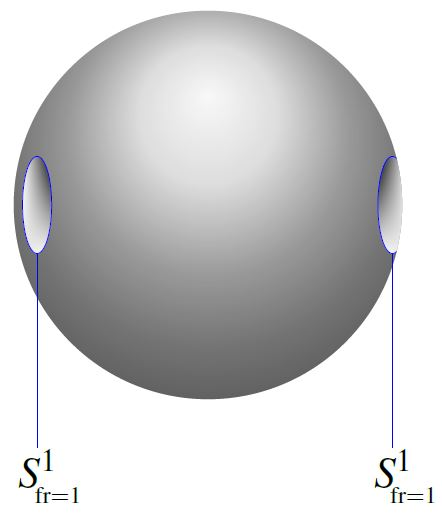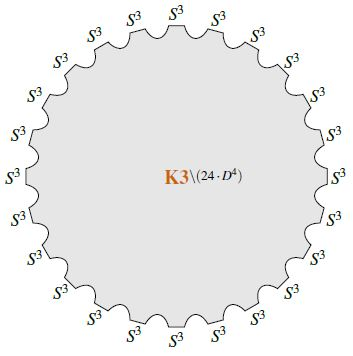# nLab homotopy groups of spheres

Homotopy groups of spheres

### Context

#### Spheres

n-sphere

low dimensional n-spheres

#### Stable Homotopy theory

stable homotopy theory

Introduction

# Homotopy groups of spheres

My initial inclination was to call this book The Music of the Spheres, but I was dissuaded from doing so by my diligent publisher, who is ever mindful of the sensibilities of librarians. (D. Ravenel 86, preface)

With all due respect to anyone interested in them, the stable homotopy groups of spheres are a mess. (J. F. Adams 74, p 204)

## Idea

The homotopy groups of spheres $\pi_{n+k}(S^n)$ are the homotopy classes of maps $S^{n+k} \longrightarrow S^n$

$\pi_{n+k}(S^n) \coloneqq [S^{n+k}, S^n] \,.$

For fixed $k$, the colimit over $n$ with respect to the suspension homomorphism

$\pi_{n+k}(S^n) \longrightarrow \pi_{n+k+1}(S^{n+1})$

over all $\pi_{n+k}(S^n)$ (called the $k$-stem) is called the stable homotopy groups of spheres (also: the “stable $k$-stem”)

$\pi_k^S = \coloneqq \underset{\longrightarrow}{\lim}_n \pi_{n+k}(S^n) \,.$

In fact, by the Freudenthal suspension theorem, the value of the $\pi_{n+k}(S^n)$ stabilizes for $n \gt k+1$ (depend only on $k$ in this range), whence the name.

The stable homotopy groups of sphere are equivalently the homotopy groups of a spectrum for the sphere spectrum $\mathbb{S}$

$\pi_k^S = \pi_k(\mathbb{S}) \,.$

The stable homotopy groups of spheres are notorious for their immense computational richness. Many of the tools of algebraic topology and stable homotopy theory were devised to compute more and more of the stable stems. This notably include the Adams spectral sequence, the Adams-Novikov spectral sequence.

## Tables

The first few stable homotopy groups of the sphere spectrum $\mathbb{S}$ are

$k =$0123456789101112131415$\cdots$
$\pi_k(\mathbb{S}) =$$\mathbb{Z}$$\mathbb{Z}/2$$\mathbb{Z}/2$$\mathbb{Z}/{24}$$0$$0$$\mathbb{Z}/2$$\mathbb{Z}/{240}$$(\mathbb{Z}/2)^2$$(\mathbb{Z}/2)^3$$\mathbb{Z}/6$$\mathbb{Z}/{504}$$0$$\mathbb{Z}/3$$(\mathbb{Z}/2)^2$$\mathbb{Z}/{480} \oplus \mathbb{Z}/2$$\cdots$

The following tables show the p-primary decomposition of these and the following stable homotopy groups.

The horizontal index is the degree $n$ of the stable homotopy group $\pi_n$. The appearance of a string of $k$ connected dots vertically above index $n$ means that there is a direct summand primary group of order $p^k$. The bottom rows in each case are given by the image of the J-homomorphism.

See at fundamental theorem of finitely generated abelian grouops – Graphical representation for details on the notation used in these table, and see example below for illustration.

(the following graphics are taken from Hatcher, based on (Ravenel 86)

$p = 2$-primary component$p = 3$-primary component$p = 5$-primary component###### Example

The finite abelian group $\pi_3(\mathbb{S}) \simeq \mathbb{Z}_{24}$ decomposes into primary groups as $\simeq \mathbb{Z}_8 \oplus \mathbb{Z}_3$. Here $8 = 2^3$ corresponds to the three dots above $n = 3$ in the first table, and $3 = 3^1$ to the single dot over $n = 3$ in the second.

The finite abelian group $\pi_7(\mathbb{S}) \simeq \mathbb{Z}_{240}$ decomposes into primary groups as $\simeq \mathbb{Z}_{16} \oplus \mathbb{Z}_3 \oplus \mathbb{Z}_5$. Here $16 = 2^4$ corresponds to the four dots above $n = 7$ in the first table, and $3 = 3^1$ to the single dot over $n = 7$ in the second and $5 = 5^1$ to the single dot over $n = 7$ in the third table.

## Examples

### 0th stem

See at Hopf degree theorem

### 1st stem

The first stable homotopy group of spheres (the first stable stem) is the cyclic group of order 2:

(1)$\array{ \pi_1^s &\simeq& \mathbb{Z}/2 \\ [h_{\mathbb{C}}] &\leftrightarrow&  }$

where the generator $ \in \mathbb{Z}/2$ is represented by the complex Hopf fibration $S^3 \overset{h_{\mathbb{C}}}{\longrightarrow} S^2$.from SS21

Under the Pontrjagin-Thom isomorphism, identifying the stable homotopy groups of spheres with the bordism ring $\Omega^{fr}_\bullet$ of stably framed manifolds (see at MFr), the generator (1) is represented by the 1-sphere (with its left-invariant framing induced from the identification with the Lie group U(1))

$\array{ \pi_1^s & \simeq & \Omega_1^{fr} \\ [h_{\mathbb{C}}] & \leftrightarrow & [S^1_{fr=1}] \,. }$

Moreover, the relation $2 \cdot [S^1_{Lie}] \,\simeq\, 0$ is represented by the bordism which is the complement of 2 open balls inside the 2-sphere.

### 3rd stem

The third stable homotopy group of spheres (the third stable stem) is the cyclic group of order 24:

(2)$\array{ \pi_3^s &\simeq& \mathbb{Z}/24 \\ [h_{\mathbb{H}}] &\leftrightarrow&  }$

where the generator $ \in \mathbb{Z}/24$ is represented by the quaternionic Hopf fibration $S^7 \overset{h_{\mathbb{H}}}{\longrightarrow} S^4$.from SS21

Under the Pontrjagin-Thom isomorphism, identifying the stable homotopy groups of spheres with the bordism ring $\Omega^{fr}_\bullet$ of stably framed manifolds (see at MFr), the generator (2) is represented by the 3-sphere (with its left-invariant framing induced from the identification with the Lie group SU(2) $\simeq$ Sp(1) )

$\array{ \pi_3^s & \simeq & \Omega_3^{fr} \\ [h_{\mathbb{H}}] & \leftrightarrow & [S^3_{fr=1}] \,. }$

Moreover, the relation $24 \cdot [S^3_{Lie}] \,\simeq\, 0$ is represented by the bordism which is the complement of 24 open balls inside the K3-manifold (e.g. Wang-Xu 10, Sec. 2.6, Bauer 10, SP 17).

Equivalently, the elements of $\pi_3^s \,\simeq\, \Omega^{fr}_3$ are detected by half the Todd classes of cobounding manifolds with special unitary group-tangential structure on their stable tangent bundle (elements of the MSUFr-bordism ring):

We have the following short exact sequence of the MSU-, MSUFr- and MFr-bordism rings (Conner-Floyd 66, p. 104)

(3)$\array{ 0 \to & \Omega^{SU}_{8\bullet+4} & \overset{i}{\longrightarrow} & \Omega^{SU,fr}_{8\bullet+4} & \overset{\partial}{ \longrightarrow } & \Omega^{fr}_{8\bullet + 3} & \simeq & \pi^s_{8\bullet+3} \\ & \big\downarrow{}^{\tfrac{1}{2}\mathrlap{Td}} && \big\downarrow{}^{\tfrac{1}{2}\mathrlap{Td}} && \big\downarrow{}^{} && \big\downarrow{}^{e_{\mathbb{R}}} \\ 0 \to & \mathbb{Z} &\overset{\;\;\;\;\;}{\hookrightarrow}& \mathbb{Q} &\overset{\;\;\;\;}{\longrightarrow}& \mathbb{Q}/\mathbb{Z} &=& \mathbb{Q}/\mathbb{Z} }$

which produces from half the Todd class of cobounding $(SU,fr)$-manifolds the KO-theoretic Adams e-invariant $e_{\mathbb{R}}$ (Adams 66, p. 39) of the boundary manifold in $\Omega^{fr}_{8k + 3} \simeq \pi^s_{8k+3}$. For $k = 0$ this detects the third stable homotopy group of spheres, by the following:

###### Proposition

(Adams 66, Example 7.17 and p. 46)

In degree 3, the KO-theoretic e-invariant $e_{\mathbb{R}}$ takes the value $\left[\tfrac{1}{24}\right] \in \mathbb{Q}/\mathbb{Z}$ on the quaternionic Hopf fibration $S^7 \overset{h_{\mathbb{H}}}{\longrightarrow} S^4$ and hence reflects the full third stable homotopy group of spheres:

$\array{ \pi^s_3 & \underoverset{ \simeq }{ e_{\mathbb{R}} }{ \;\;\longrightarrow\;\; } & \mathbb{Z}/24 & \subset & \mathbb{Q}/\mathbb{Z} \\ [h_{\mathbb{H}}] &&\mapsto&& \left[\tfrac{1}{24}\right] }$

while $e_{\mathbb{C}}$ sees only “half” of it (by Adams 66, Prop. 7.14).

## Properties

### Serre finiteness theorem

###### Theorem

(Serre finiteness theorem)

The homotopy group $\pi_{n+k}(S^k)$ is a finite group except

1. for $n = 0$ in which case $\pi_k(S^k) = \mathbb{Z}$;

2. $k = 2m$ and $n = 2m -1$ in which case

$\pi_{4m - 1}(S^{2m}) \simeq \mathbb{Z} \oplus F_m$

for $F_m$ a finite group.

### Relation to the framed bordism ring

By Thom's theorem, for any (B,f)-structure $\mathcal{B}$, there is an isomorphism (of commutative rings)

$\Omega^{\mathcal{B}}_\bullet \overset{\simeq}{\longrightarrow} \pi_\bullet(M\mathcal{B})$

from the cobordism ring of manifolds with stable normal $\mathcal{B}$-structure to the homotopy groups of the universal $\mathcal{B}$-Thom spectrum.

Now for $\mathcal{B} = Fr$ framing structure, then

$M Fr \simeq \mathbb{S}$

is equivalently the sphere spectrum. Hence in this case Thom's theorem states that there is an isomorphism

$\Omega^{fr}_\bullet \overset{\simeq}{\longrightarrow} \pi_\bullet(\mathbb{S})$

between the framed cobordism ring and the stable homotopy groups of spheres.

For discussion of computation of $\pi_\bullet(\mathbb{S})$ this way, see for instance (Wang-Xu 10, section 2) and (Putnam).

For instance

• $\Omega^{fr}_0 = \mathbb{Z}$ because there are two $k$-framings on a single point, corresponding to $\pi_0(O(k)) \simeq \mathbb{Z}_2$, the negative of a point with one framing is the point with the other framing, and so under disjoint union, the framed points form the group of integers;

• $\Omega^{fr}_1 = \mathbb{Z}_2$ because the only compact connected 1-manifold is the circle, there are two framings on the circle, corresponding to $\pi_1(O(k)) \simeq \mathbb{Z}_2$ and they are their own negatives.

### J-homomorphism and Adams e-invariant

The following characterizes the image of the J-homomorphism

$J \;\colon\; \pi_\bullet(O) \longrightarrow \pi_\bullet(\mathbb{S})$

from the homotopy groups of the stable orthogonal group to the stable homotopy groups of spheres. This was first conjectured in (Adams 66) (since called the Adams conjecture) and then proven in (Quillen 71).

###### Remark

By the discussion at orthogonal group – homotopy groups we have that the homotopy groups of the stable orthogonal group are

$n\;mod\; 8$01234567
$\pi_n(O)$$\mathbb{Z}_2$$\mathbb{Z}_2$0$\mathbb{Z}$000$\mathbb{Z}$

Because all groups appearing here and in the following are cyclic groups, we instead write down the order

$n\;mod\; 8$01234567
${\vert\pi_n(O)\vert}$221$\infty$111$\infty$
###### Theorem

The stable homotopy groups of spheres $\pi_n(\mathbb{S})$ are the direct sum of the (cyclic) image of the J-homomorphism, and the kernel of the Adams e-invariant.

Moreover,

• for $n = 0 \;mod \;$ and $n = 1 \;mod \; 8$ and $n$ positive the J-homomorphism is injective, hence its image is $\mathbb{Z}_2$,

• for $n = 3\; mod\; 8$ and $n = 7 \; mod \; 8$ hence for $n = 4 k -1$, the order of the image is equal to the denominator of $B_{2k}/4k$, where $B_{2k}$ is the Bernoulli number

• for all other cases the image is necessarily zero.

$n$012345678910111213141516
Whitehead tower of orthogonal grouporientationspinstringfivebraneninebrane
homotopy groups of stable orthogonal group$\pi_n(O)$$\mathbb{Z}_2$$\mathbb{Z}_2$0$\mathbb{Z}$000$\mathbb{Z}$$\mathbb{Z}_2$$\mathbb{Z}_2$0$\mathbb{Z}$000$\mathbb{Z}$$\mathbb{Z}_2$
stable homotopy groups of spheres$\pi_n(\mathbb{S})$$\mathbb{Z}$$\mathbb{Z}_2$$\mathbb{Z}_2$$\mathbb{Z}_{24}$00$\mathbb{Z}_2$$\mathbb{Z}_{240}$$\mathbb{Z}_2 \oplus \mathbb{Z}_2$$\mathbb{Z}_2 \oplus \mathbb{Z}_2 \oplus \mathbb{Z}_2$$\mathbb{Z}_6$$\mathbb{Z}_{504}$0$\mathbb{Z}_3$$\mathbb{Z}_2 \oplus \mathbb{Z}_2$$\mathbb{Z}_{480} \oplus \mathbb{Z}_2$$\mathbb{Z}_2 \oplus \mathbb{Z}_2$
image of J-homomorphism$im(\pi_n(J))$0$\mathbb{Z}_2$0$\mathbb{Z}_{24}$000$\mathbb{Z}_{240}$$\mathbb{Z}_2$$\mathbb{Z}_2$0$\mathbb{Z}_{504}$000$\mathbb{Z}_{480}$$\mathbb{Z}_2$

### General

Introductions and surveys include

A tabulation of stable homotopy groups of spheres is in

Original articles on basic properties:

Early computation of unstable homotopy groups of spheres $\pi_{n+k}(S^k)$ up to $n\leq 19$:

• Hirosi Toda, Composition Methods in Homotopy Groups of Spheres, Annals of Mathematics Studies Vol. 49, Princeton University Press (1962) (jstor:j.ctt1bgzb5t)

See also

### Image of the J-homomorphism

Discussion of the image of the J-homomorphism is due to

• John Adams, On the groups $J(X)$ IV, Topology 5: 21,(1966) Correction, Topology 7 (3): 331 (1968)

• Daniel Quillen, The Adams conjecture, Topology. an International Journal of Mathematics 10: 67–80 (1971)

### Formalization in homotopy type theory

For formalization in homotopy type theory see